Everyday maths 1

Start this free course now. Just create an account and sign in. Enrol and complete the course for a free statement of participation or digital badge if available.

Free course

# 4 Capacity

Now you are going to look at capacity, which can also be referred to as volume. Capacity is the maximum amount that something can contain; volume is the amount of space that a substance or object occupies. The two terms are interchangeable and can refer to the same calculation or measurement.

When you buy milk, how much is in each bottle or carton? What about when you buy juice?

Most people buy milk in cartons or bottles of one, two, four or six pints. Juice is usually sold in cartons or bottles of one litre.

Pints are an imperial measure of volume, and litres are a metric measure of volume. One litre is the same as 1,000 millilitres. Volume is the amount of space that something takes up.

To measure a very small amount, you might use a teaspoon. This is the same as 5 millilitres (ml).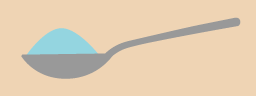Figure 27 A teaspoon

To measure larger amounts, you would probably use a measuring jug of some kind – note that measuring jugs can come in different sizes.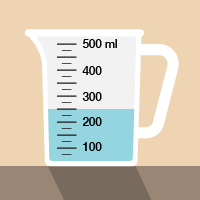Figure 28 A measuring jug

Now take a look at the following example.

## Example: Measuring liquids

If you had to measure out 350 ml of juice for a recipe, where would the liquid come to in this jug?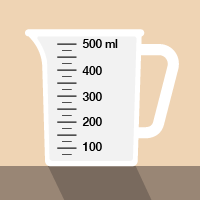Figure 29 Measuring liquids in a measuring jug

### Method

There are three marks on the jug between 300 ml and 400 ml. These mark 325, 350 and 375 ml. So you need to fill the jug to the middle mark (remember to look for the level where the liquid touches the scale):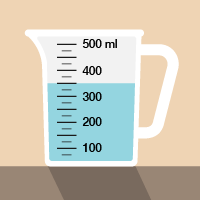Figure 30 Measuring liquids in a measuring jug (answer)

Now try the following activity. Remember to check your answers once you have completed the questions.

## Activity 9: Looking at volume

Now that you have seen the example, have a go at the following activity;

1. How much coffee or tea does a cup you usually drink out of hold? Estimate the volume first, and write down your estimate. Next, fill your cup with water and then pour the water into a measuring jug.
2. A scientist has to measure 2.8 ml of liquid in this pipette. Where should the liquid come to?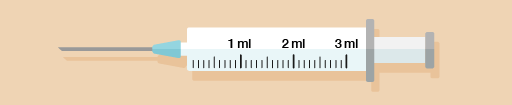Figure 31 A pipette
3. A plumber has drained water from a faulty central heating system into a set of measuring jugs. How many litres in total has the plumber drained from the system?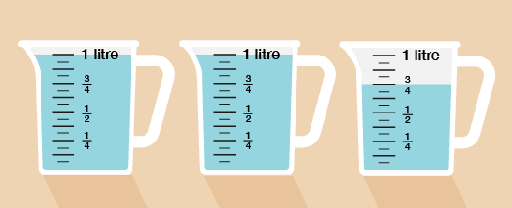Figure 32 Three measuring jugs

1. I estimated that my cup holds 400 ml. It actually holds 350 ml.
2. The divisions are marked every 0.1 ml. The pipette should look like this: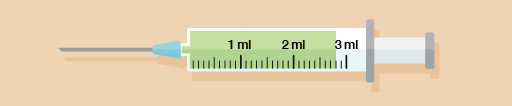3. The plumber has drained two full one-litre jugs and three-quarters of another jug, making 2.75 litres in total.
FSM_1